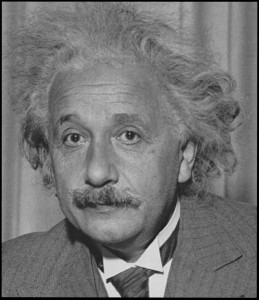# Why Can’t We Travel Faster than the Speed of Light

In a Nutshell :  We can’t travel faster than light because the faster things travel, the heavier they get.This might be news to some of you but if you’re familiar with Einsteins theory of relativity, and that most famous of equations E=mc2, you’ll understand the limitations imposed by the equation. But exactly why should it be like this ?

Science fiction wouldn’t get very far without warp speed, hyperspace or any of the other ways that spaceships travel faster than light. Unfortunately physics indicates that science fiction can never become science fact because we can never travel faster than light.

When physicists are talking about the speed of light in this context they are actually refering to the speed of light in a vacuum, such as in outer space (see side bar on slowing down the speed of light). This speed, referred to as ‘c’, is 299,792,458 m/s (327,857,019 yd/s).

# Einstein’s Famous Equation

Why is it impossible to travel faster than the speed of light in a vacuum? The answer is Einstein’s famous equation E=mc2. This equation shows how mass (m) and energy (E) are equivalent. It means that the bigger something is, the more energy is locked up inside it.

Crucially, it also means that the more energy something has, the heavier it is. Motion is a type of energy (called kinetic energy), so something that is moving is a tiny bit heavier than something that is at rest. For example if you were to throw a ball at 160.93 kph (100 mph), then it would actually get 0.000000000002 g heavier.

This is a tiny amount, but as your speed gets closer to the speed of light, the increase in mass becomes enormous. A spaceship travelling at 90 per cent of the speed of light is twice as massive as the same ship at rest. This means the engines have to work twice as hard to make it go faster. But the faster it goes the more energy it has and the more massive it becomes, and so the more energy you have to put in to speed it up. Meanwhile everything inside the ship is also getting heavier; the watch on your wrist, which used to weigh about 14 g (0.49 oz), would now weigh about 36 tonnes.

A spaceship reaching the speed of light would become infinitely heavy and would need an infinite amount of energy to move. Obviously this is impossible. This is why nothing can go as fast as – let alone faster – than the speed of light.

# A Way Around this Limitation ?

There are some ways around this problem. Some scientists think that particles with no mass such as tachyons could travel faster than light. The speed of light is their slowest possible speed. However, these are purely hypothetical particles and may not exist at all!

It might be possible to get to a distant star faster than a ray of light if you had some way of skipping the space in between. If you could fold or warp space, you could jump from one place to another without crossing the intervening distance. Perhaps it might be possible to go through a wormhole in the fabric of space-time.

Author: Robert